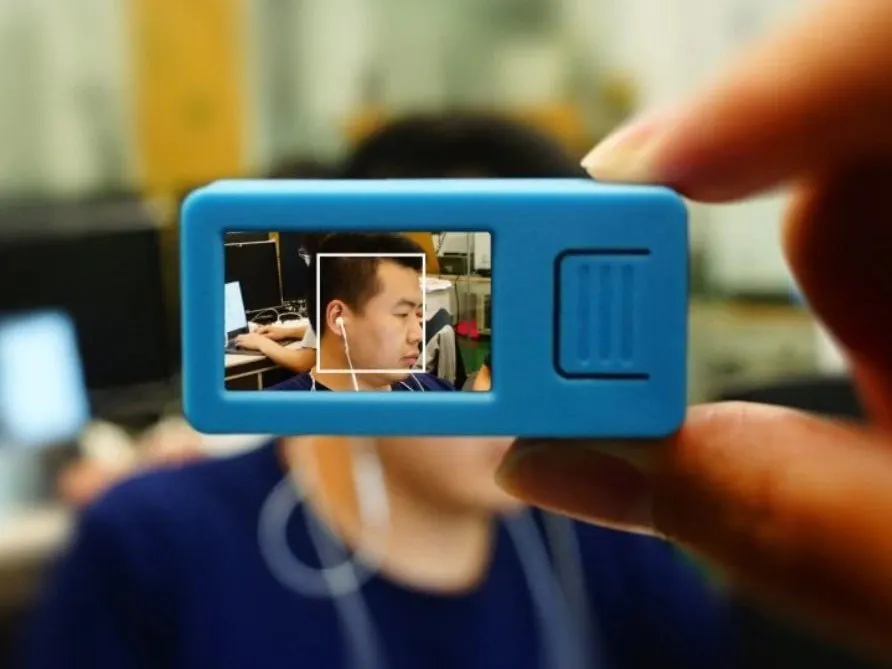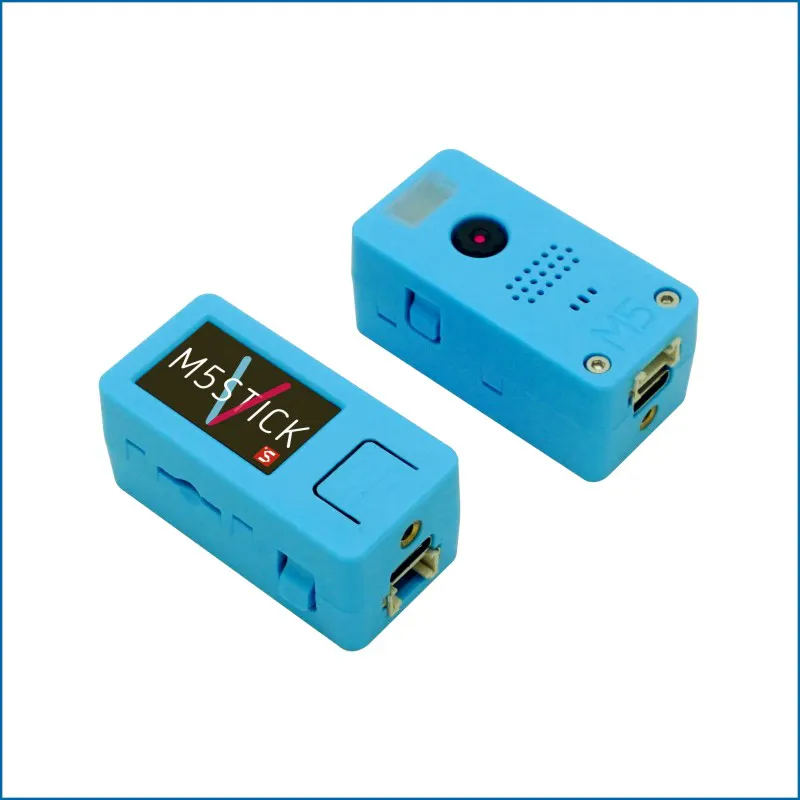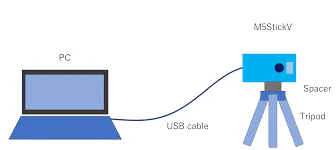# Social Distancing using Face detection (M5stickV)

Using M5stickV and its standard Face Detection Model.....

IntermediateShowcase (no instructions)1 hour9## Things used in this project

### Hardware componentsM5Stack M5StickV K210 AI Camera (Without Wifi)
×1

### Software apps and online services

 MaixPyIDE

## Schematics

### PC M5stickV Connection## Code

### demo_find_face_social_distancing

MicroPython
Maixpy IDE
```#Reuse the demo find face to create a social distancing....
#Viola variable like a umber of face inbetween
#Viola =3.5 #average distance in between
#Dist distance between two face (center of the face)
#AvgFace smallest diagonal face

import sensor
import image
import lcd
import KPU as kpu
import math
img = image.Image()
lcd.init()
sensor.reset()
sensor.set_pixformat(sensor.RGB565)
sensor.set_framesize(sensor.QVGA)
sensor.run(1)
from array import *

anchor = (1.889, 2.5245, 2.9465, 3.94056, 3.99987, 5.3658, 5.155437, 6.92275, 6.718375, 9.01025)
a = kpu.init_yolo2(task, 0.5, 0.3, 5, anchor)

while(True):
img = sensor.snapshot()
if code:
Xcor=[]
Ycor=[]
Wd=[]
Lh=[]
for i in code:
print(i.index())
print("Cx",i.x()+(i.w()/2))
print("Cy",i.y()+(i.h()/2))

Xcor.insert(i.index(),i.x()+(i.w()/2))
Ycor.insert(i.index(),i.y()+(i.h()/2))
Wd.insert(i.index(),i.w())
Lh.insert(i.index(),i.h())
n=len(Xcor)
print("face detected", n )
a = img.draw_rectangle(i.rect())
for r in range(n-1):
for c in range(0,n-r-1):

Dist = math.sqrt(((Xcor[c] - Xcor[c+1])**2) + (Ycor[c] - Ycor[c+1])**2)

uno =math.sqrt(((Wd[c] )**2) + ((Lh[c])**2))
print("Uno",uno)
dos =math.sqrt(((Wd[c+1] )**2) + ((Lh[c+1])**2))
print("Dos",dos)
AvgFace = uno
if uno >=dos:
AvgFace = dos
print("Average Face Diagonal",AvgFace)
print("Distance",Dist)
Viola =4 #average face distance in between
if ( Viola >= Dist/AvgFace ):
print("ViolatoR", c , c+1)
img.draw_string(int(Xcor[c]), int(Ycor[c]-(Lh[c]*0.25)), "X", scale=3)
img.draw_string(int(Xcor[c+1]), int(Ycor[c+1]-(Lh[c+1]*0.25)), "X", scale=3)
#lcd.draw_string(int(Xcor[c])  , int(Ycor[c])   , "XX", lcd.RED, lcd.BLACK)
#lcd.draw_string(int(Xcor[c+1])  , int(Ycor[c+1])   , "XX", lcd.RED, lcd.BLACK)
del Xcor
del Ycor
del Lh
del Wd
a = lcd.display(img)